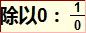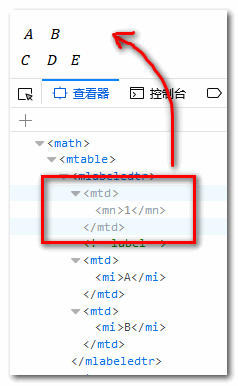# 数学标记语言MathML简介、工具及兼容

### 一、MathML简介

MathML指“数学标记语言”，是XML语言的一个子集，用来在web网页，甚至部分软件中显示数学公式。#### 1. 按类别分类的MathML表现元素

[itex]

`[itex]<mi>x</mi>[/itex]`

<mglyph>有些现存的Unicode字符是不可用的，此时就可以使用`<mglyph>`替换显示，可以理解为MathML世界中的图片元素，支持`width``height`以及`alt`等属性。例如：

```[itex]
<mi><mglyph src="my-glyph.png" alt="my glyph"/></mi>
[/itex]```

<mi>`mi`是’math identifier’的缩写，字面意思数学标识符，多指函数名，变量或者符号常量。示意（下面标识符之间无任何关联，仅仅示意语义）：

```[itex]
<mi> y </mi>
<mi> sin </mi>
<mi mathvariant="monospace"> x </mi>
<mi mathvariant="bold"> &pi; </mi>
[/itex]```

<mn>`mn`是’math number’的缩写，表示数值，支持各种数值。示意（仅示意，无关联）：

```[itex]
<mn> 0 </mn>
<mn> 1.337 </mn>
<mn> twelve </mn>
<mn> XVI </mn>
<mn> 2e10 </mn>
[/itex]```

<mo>`mn`是’math operators’的缩写，表示数学操作符，例如加减乘除，各种括号，分号等。示意：

```[itex]
<mrow>
<mn>5</mn>
<mo>+</mo>
<mn>5</mn>
</mrow>

<mrow>
<mo> [ </mo>
<mrow>
<mn> 0 </mn>
<mo> ; </mo>
<mn> 1 </mn>
</mrow>
<mo> ) </mo>
</mrow>
[/itex]```

<ms>`ms`是’math string literal’的缩写，表示一个字符串文字，这个字符串需要由编程语言和计算机代数系统来解释。默认情况下，字符串文字显示为用双引号括起来（`&quot;`）; 通过使用`lquote``rquote`属性，您可以设置要显示的自定义字符。示意：

```[itex]
<ms lquote="„" rquote="“"> abc </ms>
[/itex]```

<mspace>`mspace`是’math space’的缩写，表示空白间距，其尺寸可以通过`width``height`以及`depth`等尺寸控制。示意：

```[itex]
<mi>x</mi>
<mspace width="40px"></mspace>
<mi>y</mi>
[/itex]```

<mtext>MathML `<mtext>`元素用于呈现没有符号含义的任意文本，例如注释或注解。示意：

```[itex]
<mtext> 毕达哥拉斯定理 </mtext>
<mtext> /* 在这里注释 */ </mtext>
[/itex]```

<menclose>MathML `<menclose>`元素用来把内容封闭在指定的记号中（通过设置`notation`属性）。示意一个根号效果：

```[itex]
<msup><mi>a</mi><mn>2</mn></msup>
<mo>+</mo>
<msup><mi>b</mi><mn>2</mn></msup>
</menclose>
[/itex]```

`<menclose>`元素支持的封闭符合非常多，款式各种各样，让人大开眼界，有兴趣可以看MDN这个文档

<merror>MathML `<merror>`元素用来标记这个计算公式或者表达式是错误的，浏览器会通过边框和背景色样式加以明显区分。示意：

```<merror>
<mrow>
<mtext>除以0：</mtext>
<mfrac>
<mn> 1 </mn>
<mn> 0 </mn>
</mfrac>
</mrow>
</merror>
[/itex]```

Firefox浏览器截图效果是：<mfenced>MathML `<maction>`元素可以添加自定义的对称闭合符号（如括号），以及自定义分隔符（如逗号）。示意：

```[itex]
<mfenced open="{" close="}" separators=";;,">
<mi>a</mi>
<mi>b</mi>
<mi>c</mi>
<mi>d</mi>
<mi>e</mi>
</mfenced>
[/itex]```

<mfrac>MathML `<mfrac>``'math fraction'`，表示分数，也即是除法。示意：

```[itex]
<mfrac bevelled="true">
<mfrac>
<mi> a </mi>
<mi> b </mi>
</mfrac>
<mfrac>
<mi> c </mi>
<mi> d </mi>
</mfrac>
</mfrac>
[/itex]```

<mpadded>MathML `<mpadded>`元素用来给封闭内容增加格外的padding一遍进行位置和尺寸的调整。<mphantom>Phantom这个单词是幻影的意思。因此MathML `<mphantom>`表示当前元素是个“幻影”，也就是不可见，但是位置尺寸都保留，类似CSS世界中的`visibility:hidden`。示意：

```[itex]
<mrow>
<mi> x </mi>
<mo> + </mo>
<mphantom>
<mi> y </mi>
<mo> + </mo>
</mphantom>
<mi> z </mi>
</mrow>
[/itex]```

<mroot>MathML `<mroot>`元素表示根号。示意：

```[itex]
<mroot>
<mi>x</mi>
<mn>3</mn>
</mroot>
[/itex]```

<mrow>MathML `<mrow>`元素用于对子表达式进行分组，子表达式通常包含一个或多个运算符及其各自的操作数（例如和）。 此元素水平呈现。上面很多案例已经有示意，这里不重复演示。<msqrt>MathML `<msqrt>`元素表示开平方根。示意：

```[itex]
<msqrt>
<mi>x</mi>
</msqrt>
[/itex]```

<mstyle>MathML `<mstyle>`元素用于更改其子项的样式。例如设置颜色为茶色：

```[itex]
<mstyle displaystyle="true" mathcolor="teal">
<mrow>
<mi>i</mi>
<mo form="infix">=</mo>
<mn>1</mn>
</mrow>
</mstyle>
[/itex]```

<mmultiscripts>MathML `<mmultiscripts>`元素允许你创建一个类似张量对象。“张量”（tensor）理论是数学的一个分支学科，在力学中有重要应用。张量是一个定义在的一些向量空间和一些对偶空间的笛卡儿积上的多重线性映射，其坐标是|n|维空间内，有|n|个分量的一种量， 其中每个分量都是坐标的函数， 而在坐标变换时，这些分量也依照某些规则作线性变换。

<mover>MathML `<mover>`元素用来在表达式的上方添加重音或限制。示意：

```[itex]
<mover accent="true">
<mrow>
<mi> x </mi>
<mo> + </mo>
<mi> y </mi>
<mo> + </mo>
<mi> z </mi>
</mrow>
<mo> &#x23DE; <!--上花括号--> </mo>
</mover>
[/itex]```

<msub>MathML `<msub>`好理解，表示下标。示意：

```[itex]
<msub>
<mi>X</mi>
<mn>1</mn>
</msub>
[/itex]```

<msubsup>MathML `<msubsup>`元素表示同时上标和下标。示意：

```<math displaystyle="true">
<msubsup>
<mo> &#x222B;<!-- 积分 --> </mo>
<mn> 0 </mn>
<mn> 1 </mn>
</msubsup>
[/itex]```

<msup>MathML `<msup>`表示上标，参见下标`<msub>`<munder>MathML `<munder>`元素和`<mover>`对立，表示在表达式下方强调或者限制。示意：

```[itex]
<munder accent="true">
<mrow>
<mi> x </mi>
<mo> + </mo>
<mi> y </mi>
<mo> + </mo>
<mi> z </mi>
</mrow>
<mo> &#x23DF; <!--下花括号--> </mo>
</munder>
[/itex]```

<munderover>MathML `<munderover>`元素表示同时出现在上面和下面。示意：

```<math displaystyle="true">
<munderover >
<mo> &#x222B; <!--积分--> </mo>
<mn> 0 </mn>
<mi> &#x221E; <!--无穷--> </mi>
</munderover>
[/itex]```

<mlabeledtr>MathML `<mlabeledtr>`元素用于表示行内label标签，要么在左侧要么在右侧（由`<mtable>`元素上的`side`属性决定）。 `<mlabeledtr>`的子元素必须是`<mtd>`元素。 第一个子节点是label标签，而所有其他子节点表示行内容，并且与`<mtr>`元素的子节点相同。根据我在Firefox浏览器下的测试，应当作为label标签的第一个子节点并未显示。<mtable>MathML `<mtable>`元素可以理解为HTML届的`<table>`元素，虽然属性出入较大，但排版布局表现很类似。<mtd>MathML `<maction>`元素可以理解为HTML届的`<td>`元素，表示单元格，两者排版效果很类似。<mtr>MathML `<maction>`元素可以理解为HTML届的`<tr>`元素，表示表格行，两者排版布局效果很类似。

<maction>MathML `<maction>`元素可以添加交互行为，例如展现的是表达式，点击一下出现的是表达式计算结果。示意一个1+1=2的效果：

```[itex]
<maction actiontype="toggle">
<mrow>
<mn>1</mn>
<mo>+</mo>
<mn>1</mn>
</mrow>
<mrow>
<mo>=</mo>
<mn>2</mn>
</mrow>
</maction>
[/itex]```#### 2. 语义注释

<annotation>MathML `<annotation>`表示注解与说明。<annotation-xml>MathML `<annotation-xml>`指明函数或表达式结构。<semantics>MathML `<semantics>`表示里面含有语义化信息。

```[itex]
<semantics>
<!-- Presentation MathML -->
<mrow>
<msup>
<mi>x</mi>
<mn>2</mn>
</msup>
<mo>+</mo>
<mi>y</mi>
</mrow>
<!-- Content MathML -->
<annotation-xml encoding="MathML-Content">
<apply>
<plus/>
<apply>
<power/>
<ci>x</ci>
<cn type="integer">2</cn>
</apply>
<ci>y</ci>
</apply>
</annotation-xml>
<!-- 图像注解 -->
<annotation encoding="image/png" src="some/path/formula.png"/>
<!-- TeX注解 -->
<annotation encoding="application/x-tex">
x^{2} + y
</annotation>
</semantics>
[/itex]```

#### 其他

• 可以看到都以MathML标记都是字母`m`开头。
• 上面所有展示的MathML代码效果都可以在这个页面看到，您可以狠狠地点击这里：MathML示意demo

### 二、浏览器的兼容处理`<script src="//fred-wang.github.io/mathml.css/mspace.js"></script>`

### 三、在线生成MathML的工具介绍### 四、小众语言，总会有用的

MathML这么语言，虽然小众，但总归有用的，举例来说，我之前写了很多动画相关的文章，例如抛物线运动，矩阵变换介绍等，都需要用到数学公式，如果得到精美的自己想要的公式代码呢？经过这几天学习，我就知道该怎么玩了。